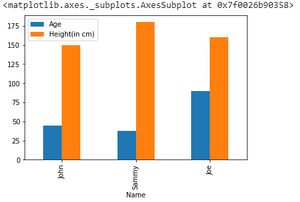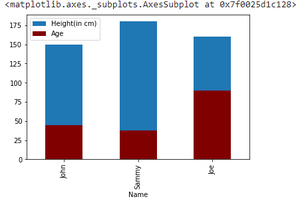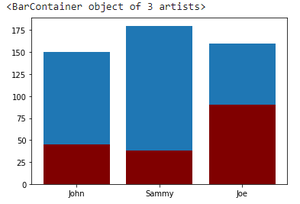# Plot Multiple Columns of Pandas Dataframe on Bar Chart with Matplotlib

• Last Updated : 24 Jan, 2021

Prerequisites:

In this article, we will learn how to plot multiple columns on bar chart using Matplotlib. Bar Plot is used to represent categories of data using rectangular bars. We can plot these bars with overlapping edges or on same axes. Different ways of plotting bar graph in the same chart are using matplotlib and pandas are discussed below.

Attention geek! Strengthen your foundations with the Python Programming Foundation Course and learn the basics.

To begin with, your interview preparations Enhance your Data Structures concepts with the Python DS Course. And to begin with your Machine Learning Journey, join the Machine Learning - Basic Level Course

### Method 1: Providing multiple columns in y parameter

The trick here is to pass all the data that has to be plotted together as a value to ‘y’ parameter of plot function.

Syntax:

matplotlib.pyplot.plot(\*args, scalex=True, scaley=True, data=None, \*\*kwargs)

Approach:

• Import module
• Pass data to plot()
• Plot graph

Example:

## Python3

 `# importing pandas library``import` `pandas as pd``# import matplotlib library``import` `matplotlib.pyplot as plt`` ` `# creating dataframe``df ``=` `pd.DataFrame({``    ``'Name'``: [``'John'``, ``'Sammy'``, ``'Joe'``],``    ``'Age'``: [``45``, ``38``, ``90``],``    ``'Height(in cm)'``: [``150``, ``180``, ``160``]``})`` ` `# plotting graph``df.plot(x``=``"Name"``, y``=``[``"Age"``, ``"Height(in cm)"``], kind``=``"bar"``)`

Output:### Method 2: By plotting on the same axis

Plotting all separate graph on the same axes, differentiated by color can be one alternative. Here again plot() function is employed.

Approach:

• Import module
• Plot first graph
• Plot all other graphs on the same axes

Example:

## Python3

 `# importing pandas library``import` `pandas as pd``# import matplotlib library``import` `matplotlib.pyplot as plt`` ` `# creating dataframe``df ``=` `pd.DataFrame({``    ``'Name'``: [``'John'``, ``'Sammy'``, ``'Joe'``],``    ``'Age'``: [``45``, ``38``, ``90``],``    ``'Height(in cm)'``: [``150``, ``180``, ``160``]``})`` ` `# plotting Height``ax ``=` `df.plot(x``=``"Name"``, y``=``"Height(in cm)"``, kind``=``"bar"``)``# plotting age on the same axis``df.plot(x``=``"Name"``, y``=``"Age"``, kind``=``"bar"``, ax``=``ax, color``=``"maroon"``)`

Output:### Method 3: By creating subplots

Another way of creating such a functionality can be plotting multiple subplots and displaying them as one. This can be done using subplot() function.

Syntax:

subplot(nrows, ncols, index, **kwargs)

Approach:

• Import module
• Create multiple subplots
• Plot on single axes

Example:

## Python3

 `# importing pandas library``import` `pandas as pd``# import matplotlib library``import` `matplotlib.pyplot as plt`` ` `# creating dataframe``df ``=` `pd.DataFrame({``    ``'Name'``: [``'John'``, ``'Sammy'``, ``'Joe'``],``    ``'Age'``: [``45``, ``38``, ``90``],``    ``'Height(in cm)'``: [``150``, ``180``, ``160``]``})`` ` `# creating subplots and plotting them together``ax ``=` `plt.subplot()``ax.bar(df[``"Name"``], df[``"Height(in cm)"``])``ax.bar(df[``"Name"``], df[``"Age"``], color``=``"maroon"``)`

Output:My Personal Notes arrow_drop_up